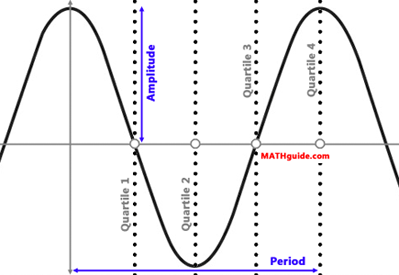Graphing Sine/Cosine: Amplitude, Period, Phase Shift, and Vertical Shift
View the Lesson | MATHguide homepage Updated August 9th, 2021Problem: f(x) = -2 + 4 cos 1/3 (x - 9°). On a sheet of paper, graph the function in degrees. Find: The function's amplitude, period, phase shift, vertical shift, and the four x-values that mark the location of its phase-shifted quartiles. Responses: amplitude =    period =    phase shift =    vertical shift =    quartile 1 =    quartile 2 =    quartile 3 =    quartile 4 =